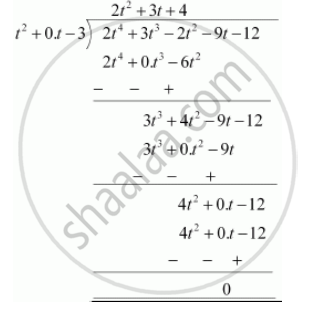# Check whether the first polynomial is a factor of the second polynomial by dividing the second polynomial by the first polynomial - Mathematics

Check whether the first polynomial is a factor of the second polynomial by dividing the second polynomial by the first polynomial

t2 – 3, 2t4 + 3t3 – 2t2 – 9t – 12

#### Solution

t2 - 3, 2t4 + 3t3 - 2t2 - 9t - 12

t2 - 3 = t2 + 0.t - 3Since the remainder is 0,

Hence, t2 - 3 is a factor of 2t4 + 3t3 - 2t2 - 9t - 12

Concept: Division Algorithm for Polynomials
Is there an error in this question or solution?
Chapter 2: Polynomials - Exercise 2.3 [Page 57]

#### APPEARS IN

RD Sharma Class 10 Maths
Chapter 2 Polynomials
Exercise 2.3 | Q 2.1 | Page 57
NCERT Class 10 Maths
Chapter 2 Polynomials
Exercise 2.3 | Q 2.1 | Page 36

Share